The discretisation of a gradient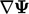is exclusively an explicit calculation using current values of. The conservative form of gradient calculation is based on a surface integral.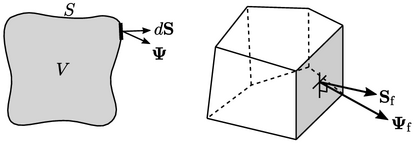From the gradient deﬁnition in Sec. 2.23 , the discretisation is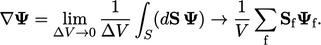(3.18)
The face value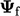is generally interpolated from cell values using the linear scheme.

### Point linear interpolation

While skewness is generally not a concern for advection discretisation, it deserves greater attention in gradient calculation. For “bad” meshes, e.g. containing elongated tetrahedral cells, the point linear scheme is often adopted to reduce skewness error.

Point linear interpolation uses: the value, calculated using linear interpolation, which corresponds to the “face point” at the intersection of the line connecting cell centres and the face; and, values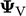at each vertex, interpolated from adjacent cells using inverse-distance weighting.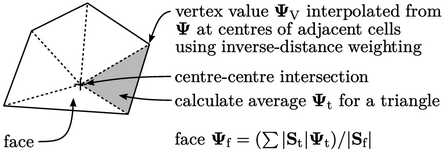The scheme breaks the polygonal face into triangles and calculates the average value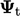at the 2 vertices and face point for each triangle, area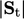. Point interpolation calculates the face value as the area-weighted average of triangle values, i.e.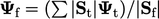.

For a given cell, a tensor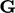is calculated by summing over faces using the inverse distance weighting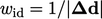, where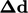is the cell centre-centre vector: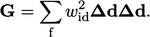(3.19)
The gradient is then evaluated using the inverse of the tensor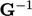and values in the neighbour (N) and current (P) cells: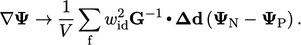(3.20)